# 20+ Fascinating Beauties That Makes Pi(π) Unique

It was finally the weekend! After my long mathematics presentation, I came home to watch my favorite tv show, Person of Interest, to de-stress. Surprisingly, the episode was about the most famous mathematical constant, pi (π), equal to the ratio of a circle’s circumference to its diameter, commonly approximated as 3.14159. Mr. Finch (the main character) acted as a substitute teacher and wrote on the chalkboard 3.1415926535. Then he asked the students, “What does this mean?”

I answered the question in my mind, thinking, “If I have a bicycle tire with a diameter of 1, then one full revolution of the bicycle tire would travel distance pi.” However, in the movie, nobody answered. Then Mr. Finch responded to the question himself, saying: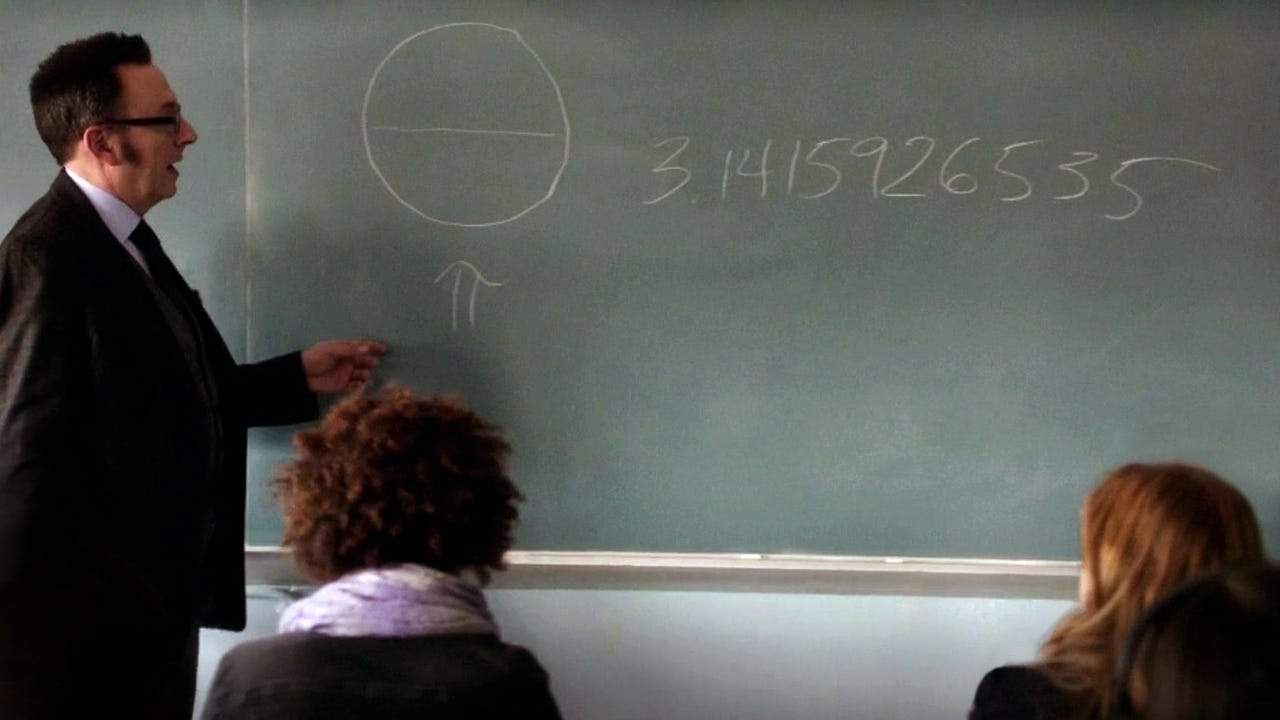Person of Interest, Season 2 Episode 11 “2 Pi R”
`Pi, the ratio of the circumference of a circle to its diameter — 3.1415926535 — is just the beginning. It keeps going forever without ever repeating, which means that contained within this string of decimals is every other number; your birth date, the combination to your locker, your social security number, etc. It’s all in there somewhere. And if you convert these decimals into letters, you would have every word that ever existed in every possible combination; the first syllable you spoke as a baby, the name of your latest crush, your entire life story from beginning to end, and everything we ever say or do. All of the world’s infinite possibilities rest within this one simple circle. Now, what will you do with that information; what it’s good for? Well, that would be up to you!`

Although that scene was actually inaccurate, I loved it. This scene is beautiful because most teachers in the world struggle to be as good and as interesting of a teacher as Mr. Finch is here. His knowledge about the subject expands the discussion beyond the textbooks and keeps the students focused throughout the lecture.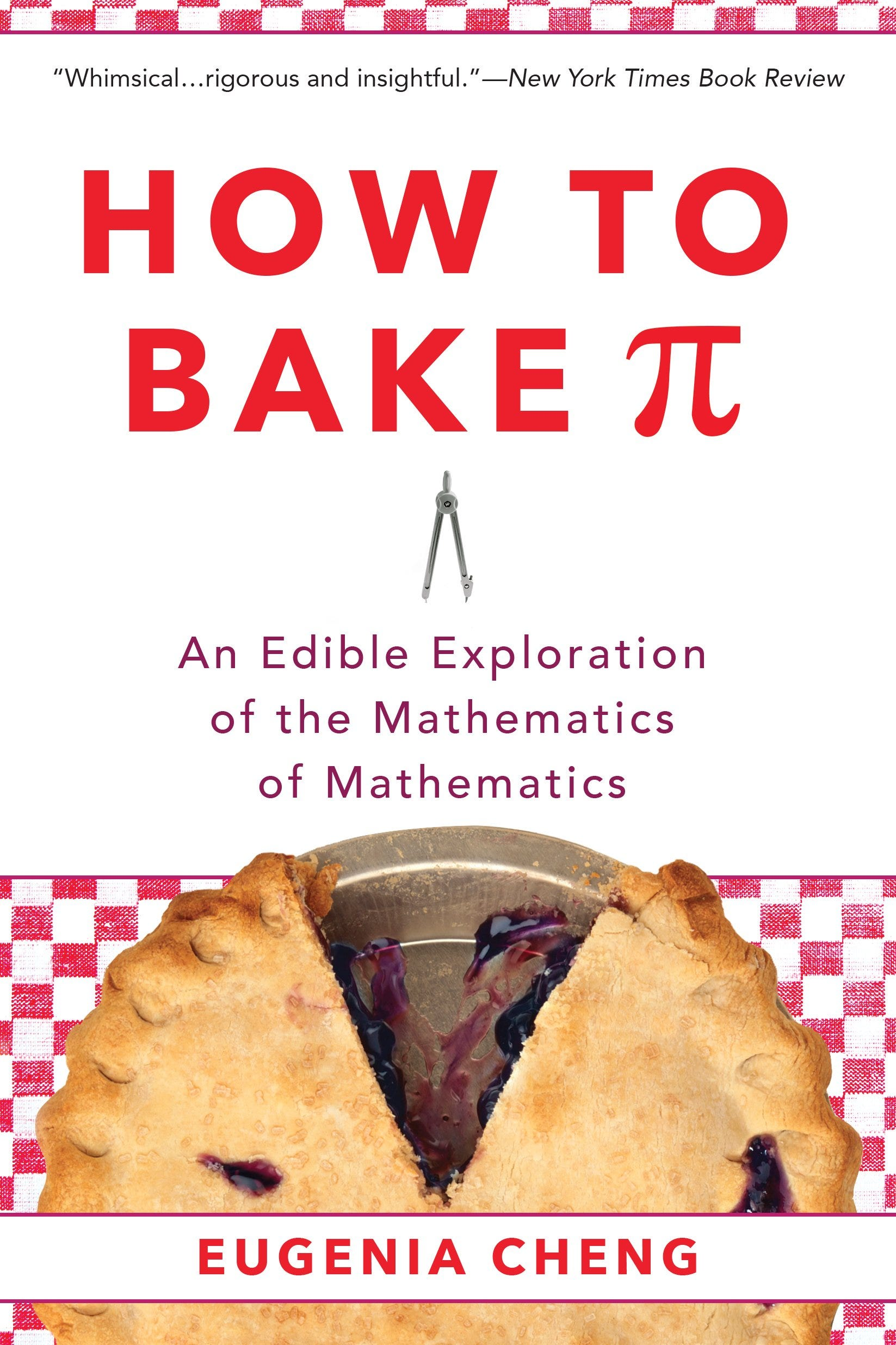Here is some beautiful books about Pi: Mandelbrot the Magnificent by Liz Ziemska | A History of Pi by Petr Beckman | How to Bake Pi by Eugenia ChengPi is the ratio of a circle’s circumference to its diameter. | Vimeo, Rebecka Taule, “Beauty In Numbers: Pi / 3.14”

Unfortunately, Mr. Finch was wrong because mathematicians have not proved that pi has the characteristic of “normality” yet. In other words, mathematicians are not sure if π contains all the finitely long permutations of digits from 0 to 9. They are not sure if every digit is used after a certain amount of time or an unlimited number of times in pi’s decimal representation.The digits of π are never-ending. | Vimeo, Rebecka Taule, “Beauty In Numbers: Pi / 3.14"
`Nobody knows what we will find in the digits of π if we keep going. For instance, when we check the first billion digits of π, we see that the digit 7 occurs almost 100 million times. This makes π an excellent random number generator because the probability of getting the digit 7 should be 1/10 or 10%. However, after some points, π may not contain the digit 7 and might instead have a non-repeating number with just two or three digits such as 010203112233000111222333… .For instance, after the first 761 digits of π, there is a famous mathematical coincidence where six nines occur in a row called Feynman point.`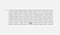Twitter, Fermat’s Library, “Feynman Point in π”

But we are sure that the digits of π keep going on forever and in random order. This randomness makes π interesting because pi’s value is finite; however, its decimal value is infinitely long. You might think this is a contradiction, but it is not. The number π is a constant number because it is the ratio of a circle’s circumference and its diameter, which are finite values. Still, we need an approximate value for π.

In 1768, Johann Lambert proved that the value π is an irrational number and cannot be written as a simple rational fraction. 22/7 is a commonly used approximation but does not contain all of the digits of π. This is because irrational numbers cannot be written as a ratio of two numbers, such as a/b. After all, irrational numbers continue to infinity and do not follow a pattern.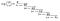Scan of formula on page 288 of Lambert’s “Mémoires sur quelques propriétés remarquables des quantités transcendantes, circulaires et logarithmiques”, Mémoires de l’Académie royale des sciences de Berlin (1768), 265–322. | Wikipedia

In 1882, Ferdinand Lindemann proved that π is a transcendental number because it is not algebraic; it is not a non-constant polynomial equation with rational coefficients.

I think we can safely say that π is transcendental because the mathematician Yasumasa Kanada found that the first trillion digits of π appear to be statistically random. His calculation took more than 600 hours. If you check the table below, you see that the event of each digit occurring is independent, and the probability of it is one-tenth of the time.

`Digit       Occurrences    0           99,999,485,134    1           99,999,945,664     2           100,000,480,057     3           99,999,787,805    4           100,000,357,857     5           99,999,671,008      6           99,999,807,503    7           99,999,818,723      8           100,000,791,469     9           99,999,854,780     Total       1,000,000,000,000`

After many years, Emma Haruka Iwao found 34.1 trillion digits of π in 2019. It took 121 days for Haruka and her computer because calculating π requires a lot of power, even for a computer. You can picture it in your mind like this; if you were to print a billion decimal values of π in normal-sized, ordinary font, it would stretch from New York to Kansas.

However, 34.1 trillion digits are still not enough to prove whether π is normal or not. Supercomputers are still crunching the numbers. If you check the graph below, you will see the number of known digits of pi, by year, since 250 B.C.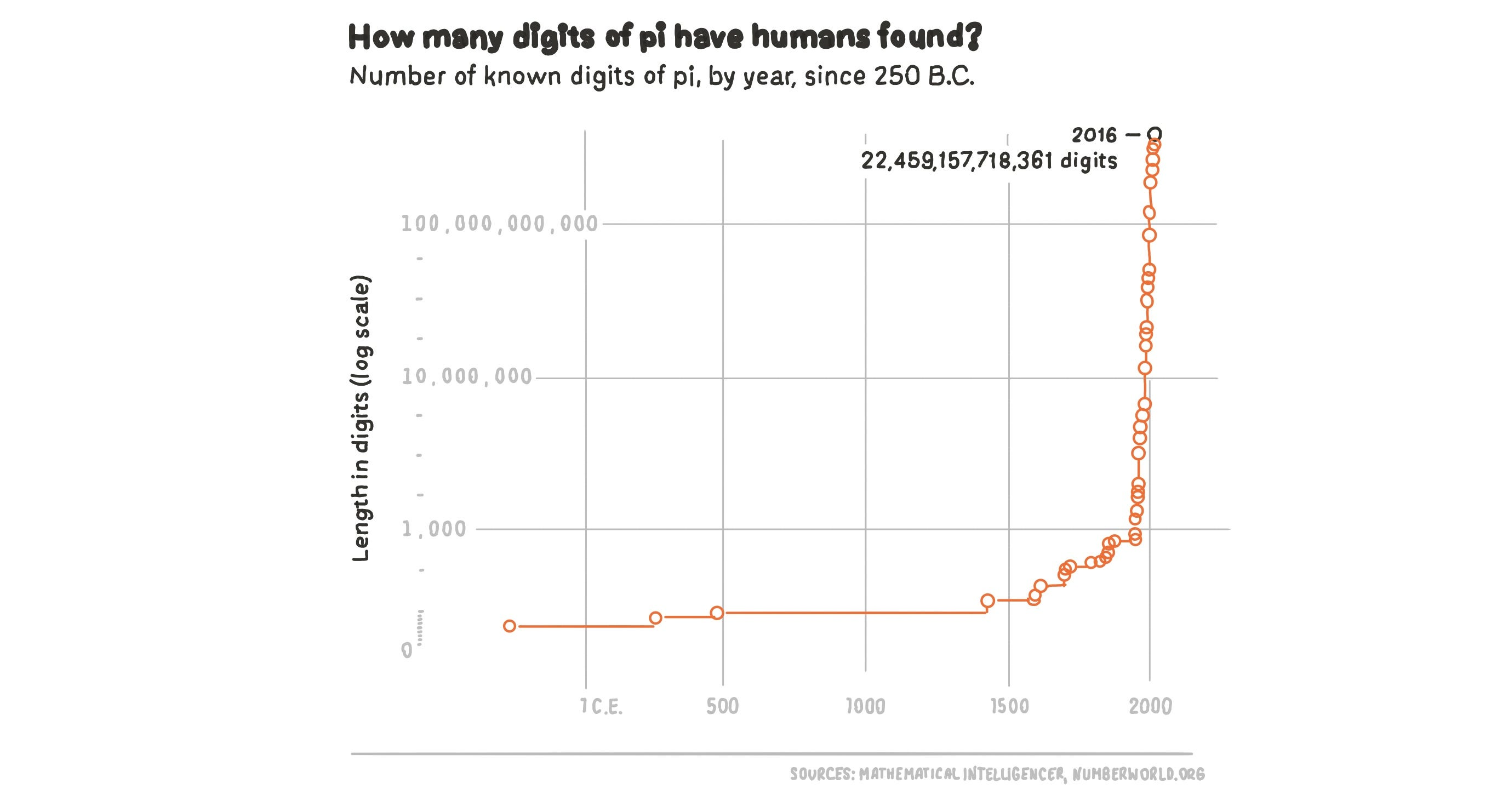Adapter from FiveThirtyEight, Graph, “Even After 31 Trillion Digits, We’re Still No Closer To The End Of Pi”

Going back to Mr. Finch, we see that he is not 100% wrong. We can find our birthdays in π easily. If you go to mypiday.com and type your birthday, it will give you the decimal place in pi. For example, my birthday occurs at the 675,097th decimal place.

`If π is a normal number, then we can say that our whole destiny is encoded in π. The pictures we will take in the future will be in π because there are binary numbers behind images. All digital products are in π. Even this article has been in π for thousands of years. Furthermore, the DNA of every creature is in π. Mr. Finch was probably right, but we are not sure yet.`

There is an interesting and artistic way to show the randomness of π. Some scientists might be happy with their tedious scatter plots, but some artists use colors for data visualization to communicate with the public. Martin Krzywinski is one such artist who found beauty and artistry in the randomness of π. He took the digits of π and gave each digit a different color. For instance, he gave three the color orange, one as red, four as yellow, etc. Then he made a beautiful poster. And if you look at it carefully, you do not see any particular pattern to the colors.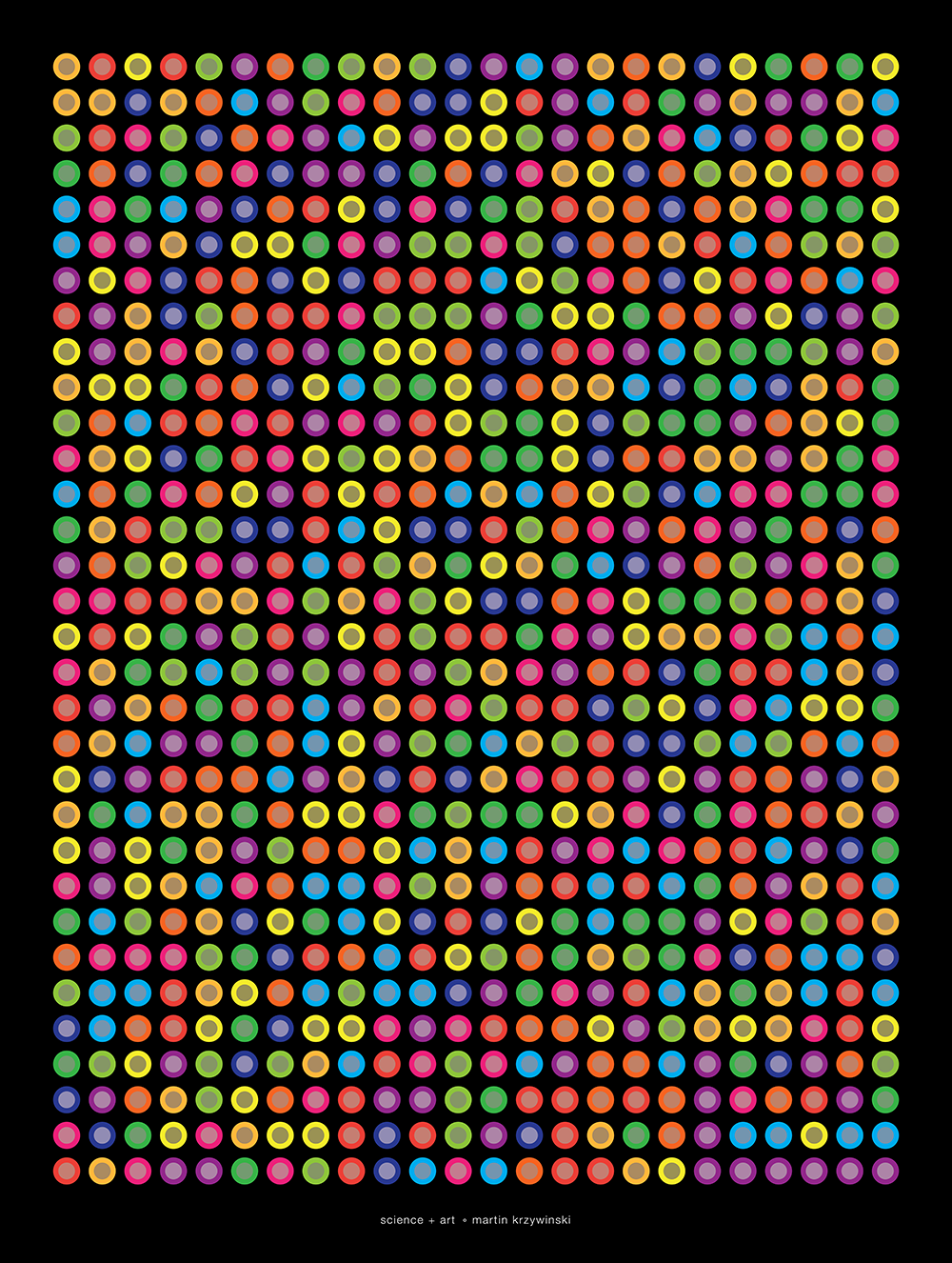Science Art by Martin Krzywinski

Besides, there are so many fascinating facts about π. It is also the most studied number in math history so far. Many people want to memorize the digits in π, not other irrational numbers. It drives people into madness and chaos. Mathematicians have been struggling to calculate π for centuries precisely.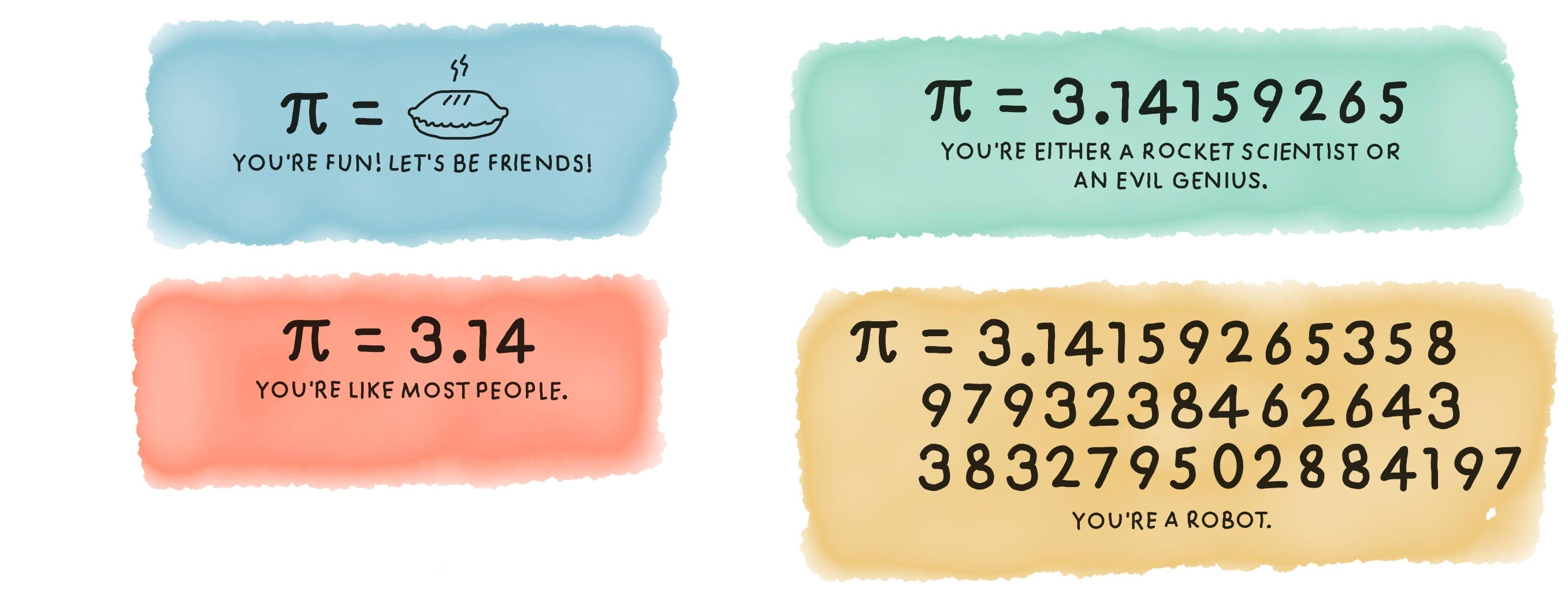What your knowledge of pi says about you? | Adapter from USA TODAY.
`So, should we stop working on π, or should we continue looking for a better approximation? Is assuming π is equal to 3.14 good enough? Or is it sufficient to use 40 digits of π to find the circumference of the Milky Way galaxy to an error less than the size of a proton? Are the first 152 digits enough to find the observable universe’s circumference at 93 billion light-years?`

There are hundreds of mathematicians who have been trying to figure out more digits of π for years. It is like trying to get to the moon and then to the next planet, and so on. But why? Why do mathematicians bother calculating any more digits? Why aren’t 34.1 trillion digits of π is not enough? Is it because π lurks in every circle?Every rotation is an expression of π. | Vimeo, Rebecka Taule, “Beauty In Numbers: Pi / 3.14”

The logical reason seems cryptic; it is because π is a beautiful source to generate random numbers. However, the real reason is that countries can show off their technology to other countries because calculating the trillion digits of pi needs a powerful computer. For instance, in the Star Trek episode “Wolf in the Fold,” Spock foils the evil computer by commanding it to “compute to last digit the value of π.” So asking a computer to compute π is called “a stress test” and may make it crash.

On the other hand, we humans are awkward creators. Staying at home and drinking tea is a beautiful activity. Still, when we get bored, we try to climb the highest of mountains, befriend a tiger, or l try to memorize the digits of π like Chao Lu, who correctly memorized the first 67,890 digits of π. We will keep doing these things because we like to understand the world around us.

`The most common mnemonic technique is to memorize the first digits of π. “May I have a large container of coffee?”`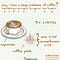“May I have a large container of coffee?” | Source: Abakcus’ Twitter

On September 12, 1962, John F. Kennedy gave a speech about the space program. He said:

`There is no strife, no prejudice, no national conflict in outer space as yet. Its hazards are hostile to us all. Its conquest deserves the best of all mankind, and its opportunity for peaceful cooperation may never come again. But why, some say, the moon? Why choose this as our goal? And they may well ask why climb the highest mountain? We choose to go to the moon. We choose to go to the moon in this decade and do the other things, not because they are easy, but because they are hard because that goal will serve to organize and measure the best of our energies and skills, because that challenge is one that we are willing to accept, one we are unwilling to postpone, and one which we intend to win, and the others, too.`

We are inevitably connected to the past, and π is a thread that’s gone through all of human history. That’s why we can say that as long as there are people, there’s always going to be somebody who wonders what’s next. And I assure you that somewhere in the world, there is a mathematician or scientist using π for something important for our universe because π is still the mysterious constant of nature.

# Finding Pi

The previous statement is utterly true because there has always been someone who works on π. Mathematics is as old as civilization. The human race has studied π for almost 4000 years. When the last mammoths were going extinct, people were exploring π. As far as we know, Archimedes from ancient Greece was one of the first humans who calculated π. He was most likely helping wheel makers. But how did he estimate the value of π?

Firstly, he saw that all polygons as a circle. According to Archimedes, if you keep increasing the number of a polygon’s sides, you would get closer to the perfect circle. In other words, a pentagon is more circle than a square, but a hexagon is more circle than a pentagon, and so on. Thus, the legendary mathematician Archimedes defined a circle as a regular polygon with an extremely large number of sides more than two thousand years ago.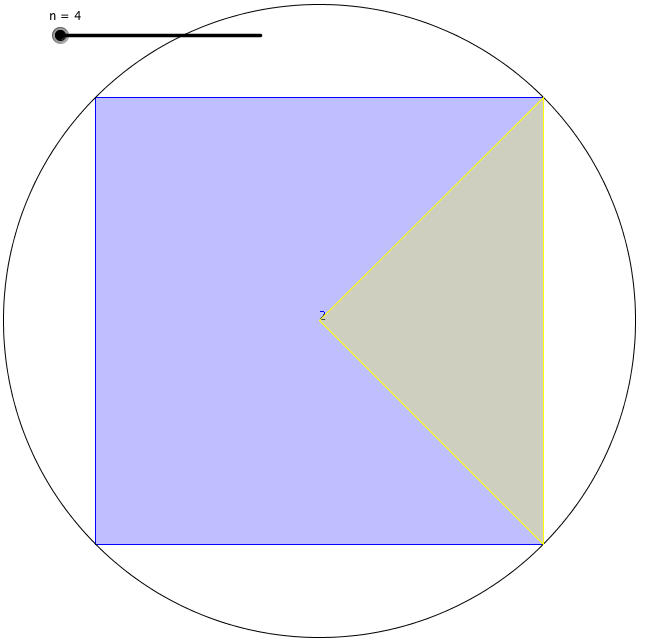Polygons inscribed in the unit circle [Forbes]
`His definition is helpful because measuring a curved surface was hard to do accurately. He found a way to find the circumference of a circle. First, he drew a square with its corners touching the perimeter of a circle and found the inscribed square’s perimeter. Secondly, he drew another square with its sides touching the circle’s perimeter and found the perimeter of the circumscribed square. He concluded that the circle’s circumference had to lie somewhere between the value of those two perimeters of squares.However, using this method, the difference between those two values was pretty big when he used squares. So, he drew pentagons to see the upper and lower bounds of the circumference of the circle. He got a smaller range of bounds at that time. After that, he kept increasing the polygon’s number of faces that he was drawing inside and outside the circle. Each time he did this, his estimation was getting more accurate. Archimedes worked hard and got up to a 96 sided regular polygon called an enneacontahexagon until he got exhausted. The lower and upper bound that he found at that time were 3.1408 and 3.1429. Thus, he calculated π to two decimal places.`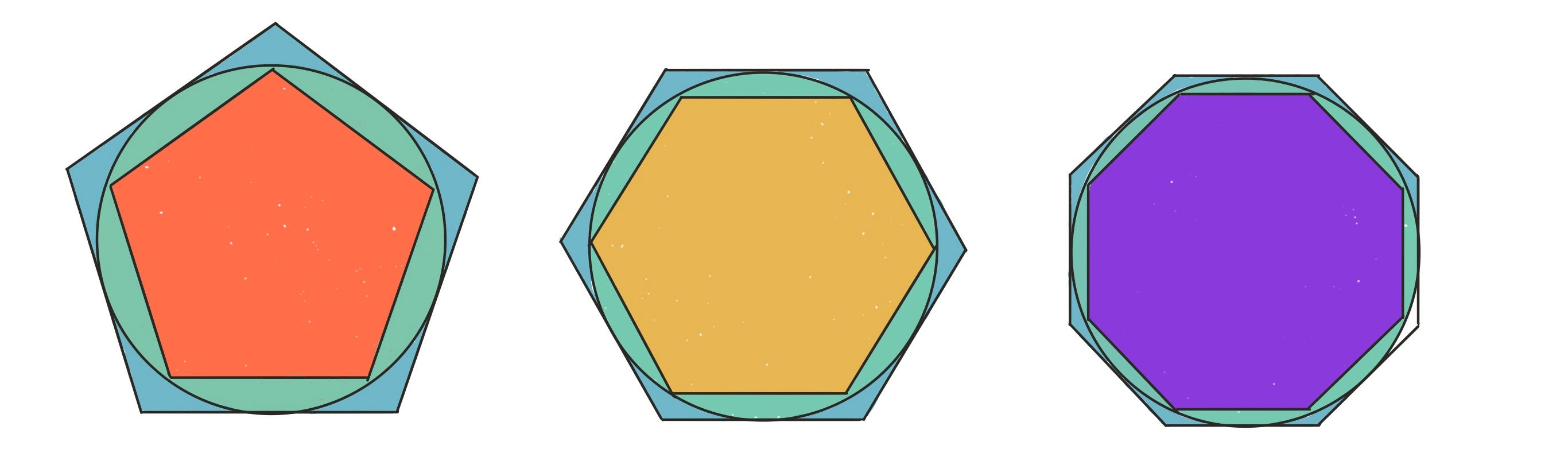Archimedes’ method for calculating π. Working with a 96-sided polygon, Archimedes computed π to an accuracy of one part in 10,000. | Adapter from NCJ.

Archimedes’ method needed improvement because his life span would not be long enough to find the other digits of π by hand. Mathematicians are required to discover more efficient formulas and new techniques.

Before mathematicians could do this, they needed to wait for Al-Khwarizmi to discover Algebra. In the beginning, people were using signs for digits. For instance, let’s say you and your neighbor have 75 horses together, and you have 35 horses. You need to find the number of horses your neighbor has. Without algebra, the solution will take a long time. But after the discovery of algebra, we just use equations to solve the problems. In this specific example, we can write 75 = x + 35, where x is the number of your neighbor’s horses. Writing such an equation and using a variable instead of a number was revolutionary to the classical world. Algebra allowed for much easier calculations across all of mathematics.

The adoption of algebra by great mathematicians inspired a whole new way of looking at the world. The next significant jump in calculating π was the invention of calculus. After that, mathematicians started working on infinite series.

`An infinite series is an expression with numbers added together with one after the other until infinity, and sometimes these endless series converge to a particular value.`

There are many methods available now to calculate π. Gottfried Leibniz found π in infinity. James Gregory found the equation below for π. He was working on one of the astonishing infinite series for the inverse tangent function below. He added infinitely many small numbers together and found π.

He put x = 1 into the inverse-tangent series. He showed us the further we go, the closer to the estimation of π we get. However, in order to get ten digits of π, we need to write about 5 billion fractions to add.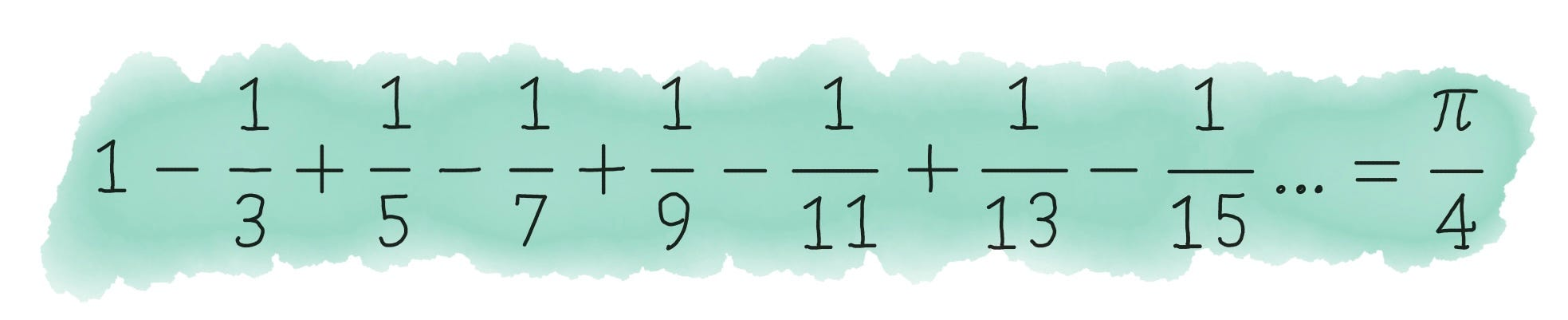The Gregory series is a pi formula found by Gregory and Leibniz and obtained by plugging x=1 into the Leibniz series.
`Borwein Integals are pretty interesting. We can see a beautiful pattern of π in those integrals. Unfortunately, the pattern eventually breaks down.`

Another great mathematician, Leonhard Euler - who officially adopted the Greek letter “π” as a symbol to represent the value- found a more efficient equation for π when he was 28. The character became iconic. Euler’s π equation calculates an infinite sum. The Basel Problem was named after him.

Euler also used π to write another beautiful equation, Euler’s Identity. I have a whole article dedicated to it, which you can read below.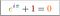Euler’s Identity relates five of the most common constants in mathematics.Alto Tolino’s beautiful art work, Identität. | Source: altotolino.com

Although imaginary numbers are not real, π can make imaginary numbers real. If you i^i into a calculator you will get 0.2078795763507619085469… . The proof is totally related to π. Below, you can see why i^i is real.

Thanks to the genius mathematician Ramanujan’s obsession for π, we have many new formulas to find π. When he arrived at Cambridge, he brought a notebook in which there were 400 pages of formulas to find π.

After the mechanical computers’ invention, mathematicians used Leibniz’s, Euler’s, and Ramanujan’s infinite series to calculate a trillion decimal digits of π. Without a supercomputer, finding the digits of π that far would be difficult. For example, the mathematician William Shanks managed to calculate the first 707 digits of π by hand, but unfortunately, he had made a mistake after the 527th place.

## Pi is everywhere.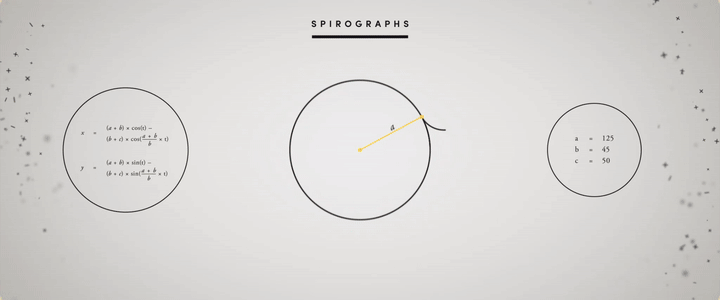Spirographs are mathematical patterns where different rotational variables generate different outcomes. | Vimeo, Rebecka Taule, “Beauty In Numbers: Pi / 3.14”

Children start learning about π when they are in 7th grade and use it until they graduate from college. Even after that, most people use π again when their children go to school. Pi appears everywhere in the universe and every time in our lives. It is literally woven into our universe; the orbits of planets, the electromagnetic waves, rivers, the colors of auroras, the structure of DNA, the Great Pyramid of Giza…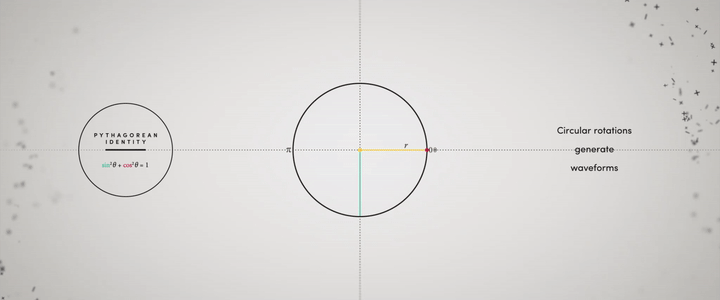π is part of the trigonometric sine and cosine functions. | Vimeo, Rebecka Taule, “Beauty In Numbers: Pi / 3.14”

Since anything involving a circle or a sphere is about π, if a scientist wants to describe the structure of the universe or finds the relationship between planets, he/she needs to use π. Circles appear throughout the natural world, whether it’s a soap bubble or the moon in the night sky. This explains why mathematics is essential in all areas of the sciences. Pi helps us to see the mathematical ideas underlying diverse physical processes.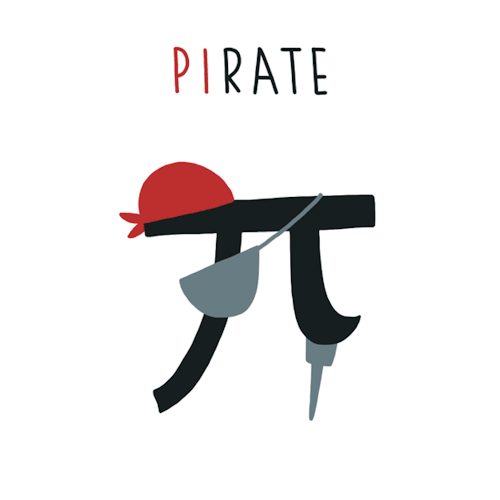A Gif showing the clever play on the letters “Pi.” | Source: Cindy Suen

## The Connection Between Pi & Sinuosity of Rivers

Pi has a direct relationship with rivers on Earth. But how? To figure this out, we need to measure the length of a river in two different ways.

`Assume that we know the starting and ending point of the river. First, we need the actual length to see how bendy the river is. In other words, the exact length is the distance that you need to swim from the beginning point to the ending point. This whole length will be “L.” Second, we need to find a straight length. In other words, this time, we need to fly from the beginning to the end. And this direct route will be a lowercase “l.” Now we can write the formula for the sinuosity by dividing L by l. The sinuosity is a ratio and measures how bendy the river is.`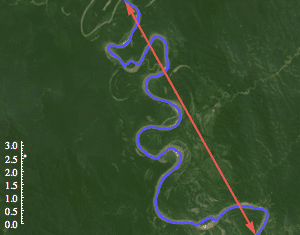The ratio is often found to converge to (but rarely exceed) 3.14, roughly pi. | Source: matthen

What is important here is there’s no limit to how high sinuosity can be. The river can be really bendy. However, Hans-Henrik Stølum proved that the average sinuosity of rivers around the world is π. If you find all the rivers’ sinuosity and take the average sinuosity, you should get π.

`There is another interesting fact about sinuosity. Rivers can be very bendy at some points. We would expect a high sinuosity. But suddenly, those rivers become straight and make the sinuosity equal π. So, it is hard to find a river’s sinuosity equal to 7 because of fluid dynamics. Mathematicians found the highest sinuosity to be around 3.5 and the lowest sinuosity around 2.7.`

Rivers can start acting very chaotic after some time. Then they suddenly go back to normal. At the extremely bendy point, rivers cut off after the bend point and make a shortcut to become straight again. This phenomenon is known as an oxbow lake, which controls the sinuosity of rivers. This keeps the sinuosity of a river around π.Time lapse of a river changing course. | Source: Reddit

## Pi in Space

There is a mathematical order inherent in our universe. For instance, to understand our solar system, we need π. We know that our planet moves in front of its host star. And the light comes from host stars. To talk about that light, we need to know how big the host star is. In other words, we need the surface area of the host star. The formula for a sphere’s surface area is 4πr², with r being the star’s radius. The size of a planet also helps scientists to guess whether it is habitable or not.For every 8 Earth orbits, Venus orbits the Sun 13 times. | Vimeo, Rebecka Taule, “Beauty In Numbers: Pi / 3.14”

Another good example to show the relationship between π and the universe is electrostatic force, which is the force between two electric charges. An electron exerts a force in all directions and forms a sphere field. Electrons also interact with each other on an electric field. To figure out that interaction, we need to find the surface area of spheres, where again π comes up.

There is also a connection between π and gravity. If you have had a chance to see Einstein’s field equations, you might notice that π is there too.

The formula above calculates how objects with a large mass, such as stars and galaxies, can curve space and time with their gravity. Einstein says that just like a ball sitting on a bedsheet, any form of momentum and energy can also curve space-time around it. In other words, the formula is saying:

`Gravity = 8 x π x Energy & Momentum`

So π is the part of the gravity, energy, and momentum of the universe and all objects in it. Not any of the other irrational numbers. If you take the square root of the gravity of Earth, you almost get π. I don’t think this is a coincidence.

`√9.8 = 3.1305`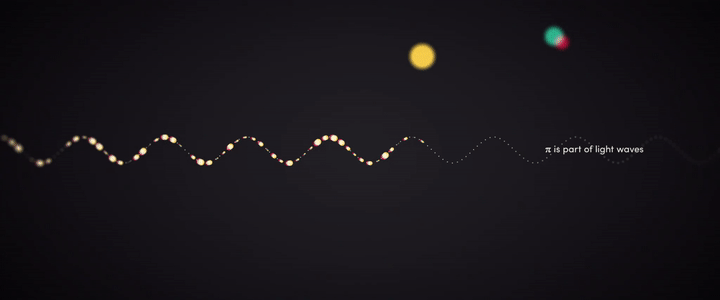π is part of light waves. Waves create color. Waves create sound. Waves create motion. | Vimeo, Rebecka Taule, “Beauty In Numbers: Pi / 3.14”

## Finding Pi in Nature

Infinite series are not the only way to find π. There are some cool and fun activities you do to estimate π on your own. One of them is called the Monte Carlo method.

`Assume that you are working on a 1x1 grid. You are generating pairs between 0 and 1 to plot the points on a coordinate plane. If you keep plotting the points, you will see that the distance of some points to the origin will be less than 1, and some of them will be greater than 1. After some point, you will see that you are getting a quarter circle. If you find the area of that quarter circle, it will be almost π/4. There is an example below of 1,000 points. You can try it from here.`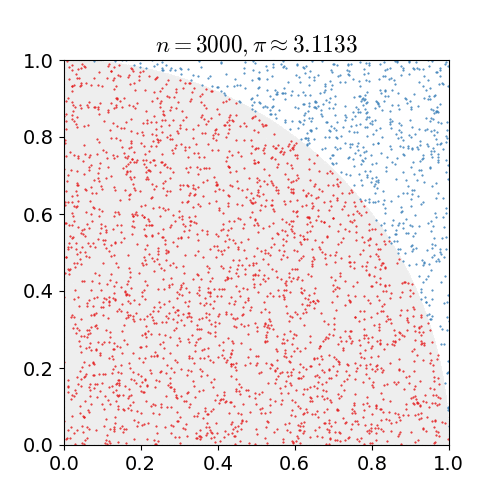Monte Carlo Simulation | Source: Wikimedia Commons
`If you don’t want to deal with computer programming, you can use just a pencil and paper to do this. You just need to draw a circle with a radius of 1 and then draw a square around the circle. The area of the square has to be four because the diameter of the circle is 2. Now, if you take your pencil and close your eyes and put random dots on the paper many times, eventually, the percentage of times your dot landed inside the circle will approach π/4. So you can feel like Archimedes here.`

## Buffon’s Needle

When there was no internet, kids used to play b tossing a coin on the floor and seeing whether the coin crosses a line or not. A French philosopher and mathematician, Georges-Louis Leclerc, decided to determine the probability that the coin would cross a line. Remarkable idea!

He started by dropping a needle on a lined sheet of paper and determining the probability of the needle crossing one of the lines on the paper. Then he tried his experiment with many needles many times. He got a remarkable result. The probability was directly related to the never-ending number pi value because two times the number of needles he dropped divided by the number of needles crossing a line was almost equal to pi all the time. So he made a formula:

`P: the probability | n: the number of needles | c: the number of needles crossing a line. Then; P = 2n/c`

After Leclerc, an Italian mathematician, Mario Lazzarini, threw needles almost 4000 times to perform this experiment. He got pi with perfect accuracy. He got to the first six decimal places of π.

If you don’t believe me, you can throw thousands of toothpicks and test the experiment yourself, or you can check the Monte Carlo simulation below to see. The gif shows the π estimation with a different number of toothpicks.Throwing 1000 needles to estimate π.| Source: Reddit

## The Pi Bicycle

In 2017, two good friends, Martijn Koomen and Tadas Maksimovas designed a fully functional pi bicycle built in the shape of the mathematical symbol, π.The Pike Bike | Source: Tadas Maksimovas

## The Pi Day

After a long history of studying π, people decided to organize an official celebration of π on March 14. Since 1988, people celebrate the magical constant on March 14. There is an interesting coincidence that Albert Einstein was born on Pi Day, March 14, 1879. Einstein also published his theory of general relativity on Pi Day.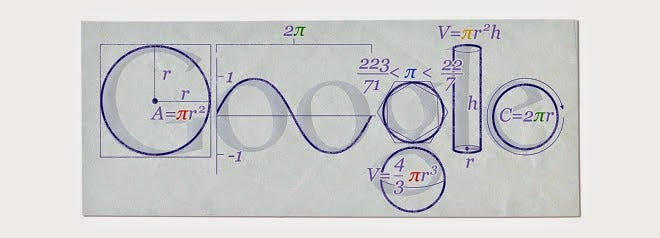The Google logos for Pi Day.

To summarize, mathematics is a language that is inscribed in the brains of all human beings. Pi is just a word in that language. John F. Kennedy knew that the moon was not infinitely far away, and he got there. I believe that one day the great mathematicians will reveal all the mysterious digits of π.

I wish Finch had been my teacher when I was a student.

## However, Mathematics

Life is good for only two things, discovering mathematics…

Written by

## Ali

Math Teacher. Content Curator. Soccer player. Maradona fan. Mostly write about the lectures I love to learn better. alikayaspor@gmail.com

## However, Mathematics

Life is good for only two things, discovering mathematics and teaching mathematics.

Written by

## Ali

Math Teacher. Content Curator. Soccer player. Maradona fan. Mostly write about the lectures I love to learn better. alikayaspor@gmail.com## However, Mathematics

Life is good for only two things, discovering mathematics and teaching mathematics.

## More From Medium

Medium is an open platform where 170 million readers come to find insightful and dynamic thinking. Here, expert and undiscovered voices alike dive into the heart of any topic and bring new ideas to the surface. Learn more

Follow the writers, publications, and topics that matter to you, and you’ll see them on your homepage and in your inbox. Explore

If you have a story to tell, knowledge to share, or a perspective to offer — welcome home. It’s easy and free to post your thinking on any topic. Write on Medium